# JavaScript 中的樹型資料結構

Tree 是一種有趣的資料結構，它在各個領域都有廣泛的應用，例如:

• DOM 是一種樹型資料結構
• 我們作業系統中的目錄和檔案可以表示為樹
• 家族層次結構可以表示為一棵樹

## 引言

```js function Node(value){ this.value = value this.left = null this.right = null } // usage const root = new Node(2) root.left = new Node(1) root.right = new Node(3)```

```js 2 / \ / \ 1 3 / \ / \ null null null null```

• 廣度優先遍歷
• 深度優先遍歷

## 廣度優先搜尋/遍歷（BFS）

```js 2, 1, 3```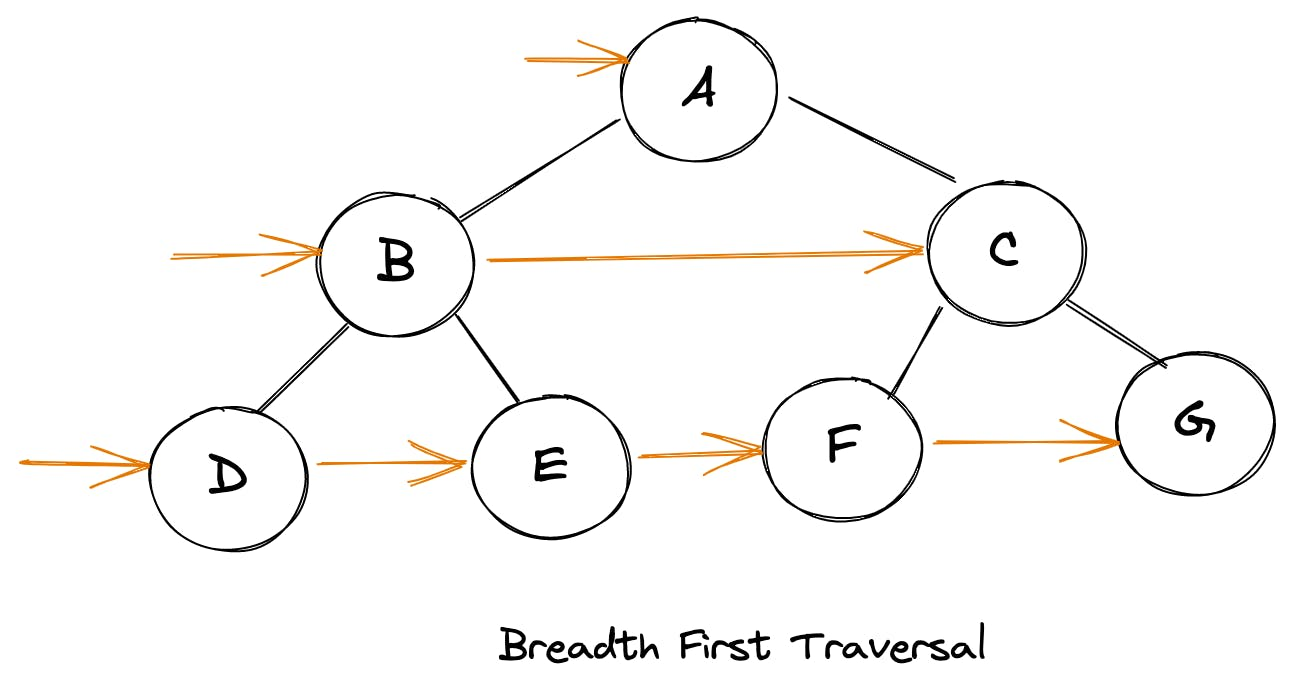• 初始化一個包含 root 的佇列
• 從佇列中刪除第一項
• 將彈出項的左右子項推入佇列
• 重複步驟2和3，直到佇列為空

```js function walkBFS(root){ if(root === null) return

const queue = [root] while(queue.length){ const item = queue.shift() // do something console.log(item)

``````  if(item.left) queue.push(item.left)
if(item.right) queue.push(item.right)
``````

} } ```

```js function walkBFS(root){ if(root === null) return

const queue = [root], ans = []

while(queue.length){ const len = queue.length, level = [] for(let i = 0; i < len; i++){ const item = queue.shift() level.push(item) if(item.left) queue.push(item.left) if(item.right) queue.push(item.right) } ans.push(level) } return ans } ```

## 深度優先搜尋/遍歷（DFS）

```js root node -> left node -> right node // pre-order traversal left node -> root node -> right node // in-order traversal left node -> right node -> root node // post-order traversal```

### 前序遍歷

```js root node -> left node -> right node```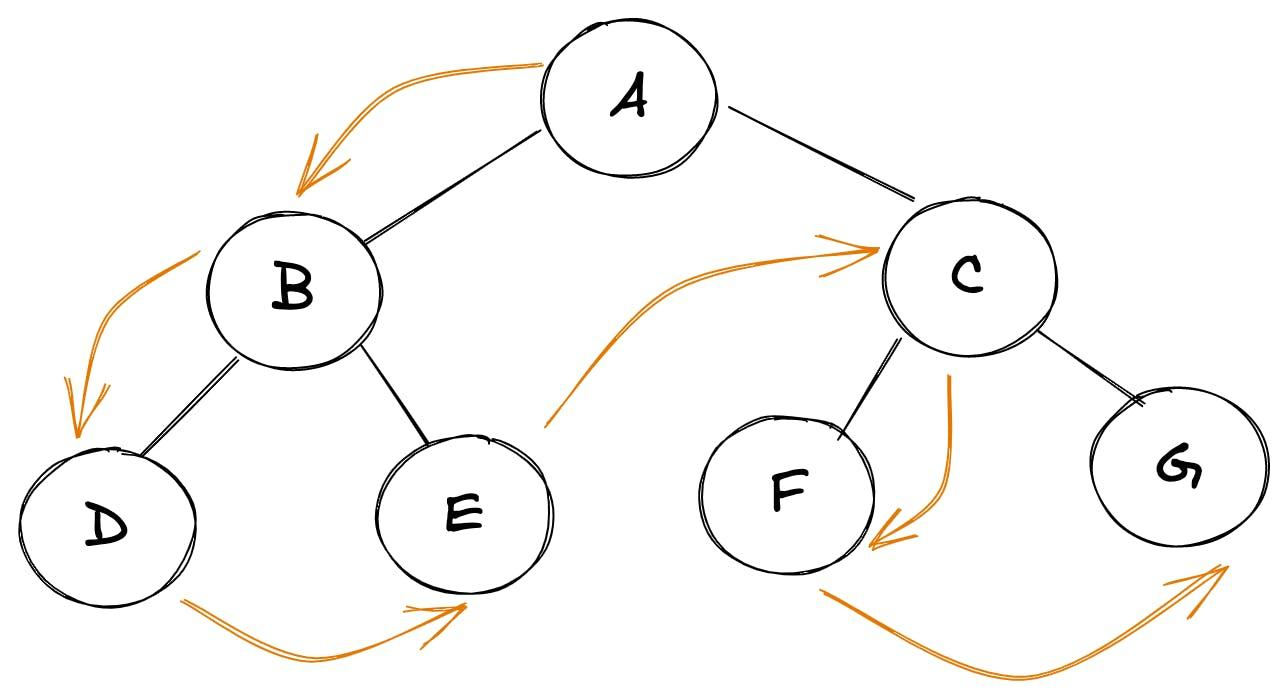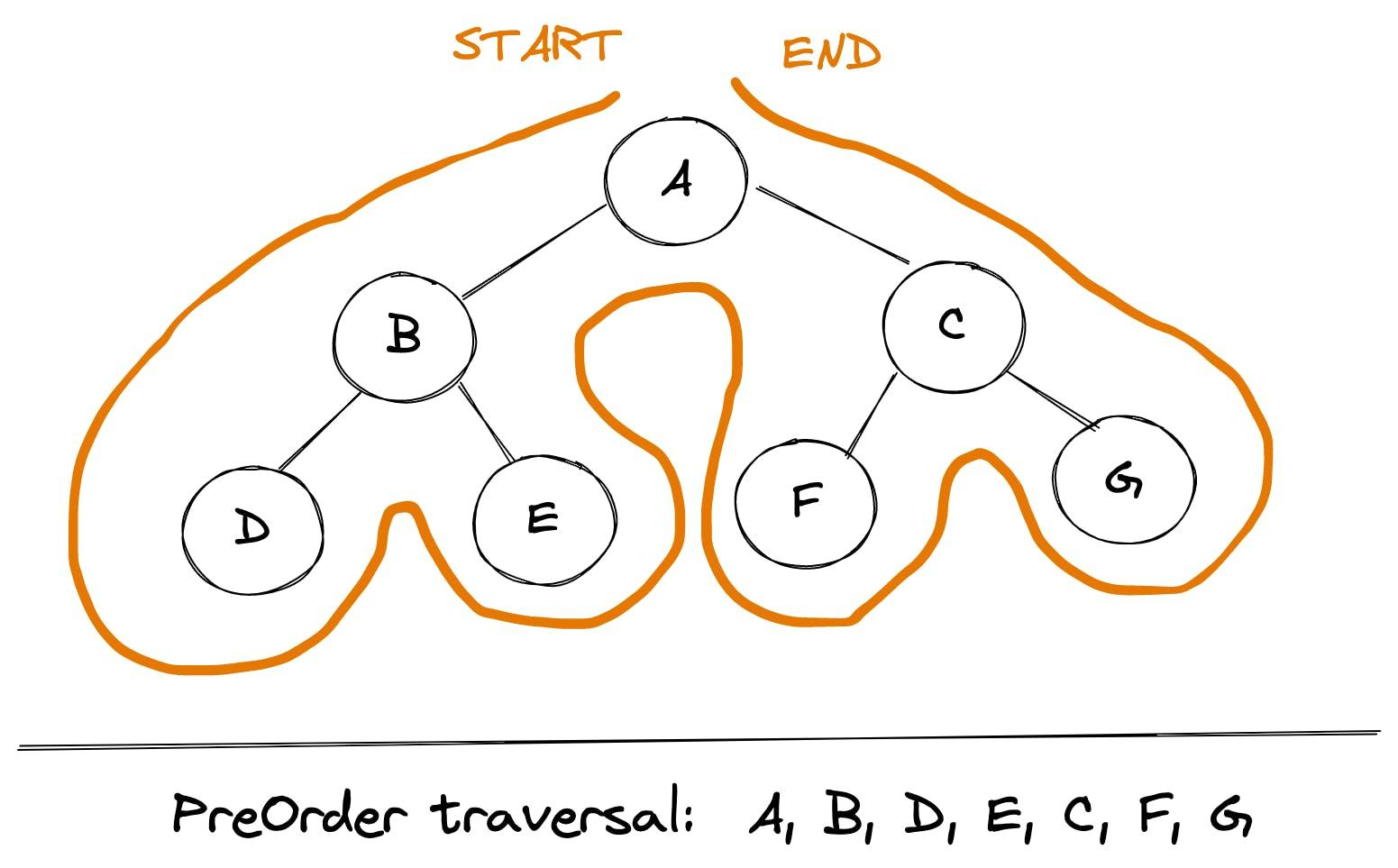```js function walkPreOrder(root){ if(root === null) return

// do something here console.log(root.val)

// recurse through child nodes if(root.left) walkPreOrder(root.left) if(root.right) walkPreOrder(root.right) } ```

```js function walkPreOrder(root){ if(root === null) return

const stack = [root] while(stack.length){ const item = stack.pop()

``````  // do something
console.log(item)

// Left child is pushed after right one, since we want to print left child first hence it must be above right child in the stack
if(item.right) stack.push(item.right)
if(item.left) stack.push(item.left)
``````

} } ```

### 中序遍歷

```js left node -> root node -> right node```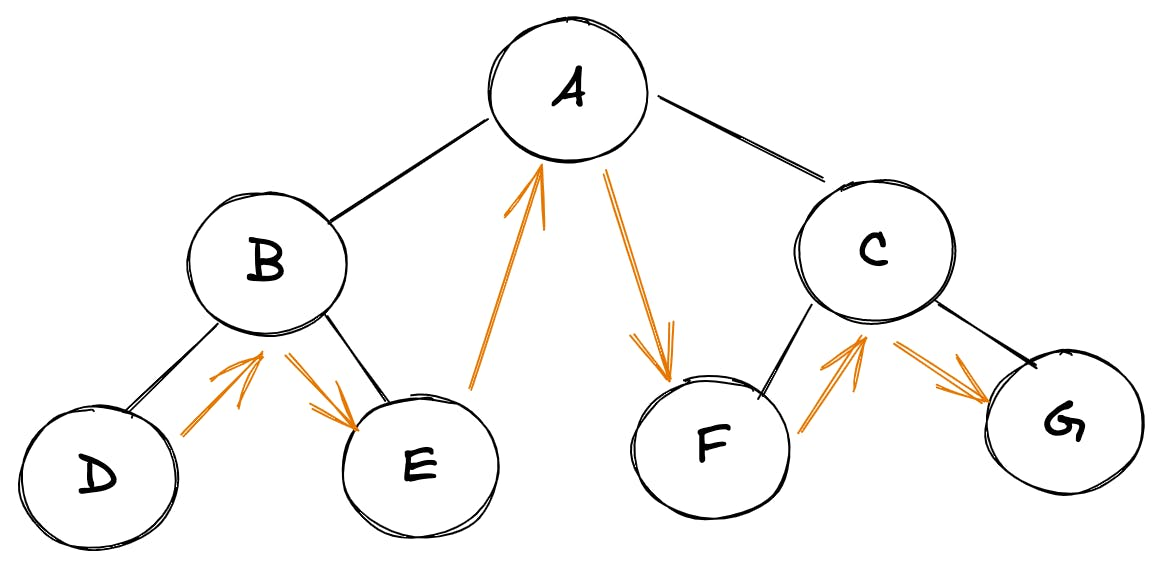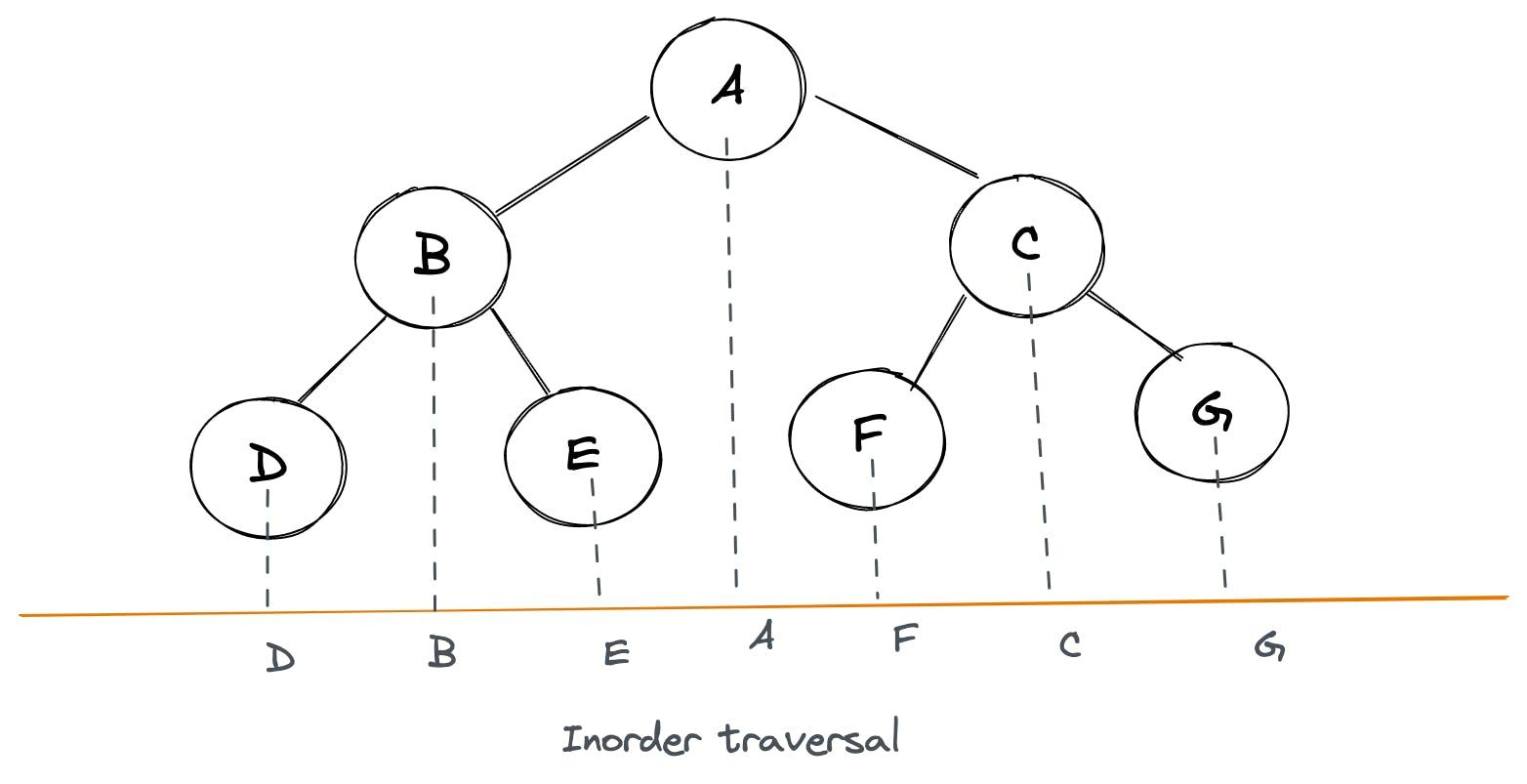```js function walkInOrder(root){ if(root === null) return

if(root.left) walkInOrder(root.left)

// do something here console.log(root.val)

if(root.right) walkInOrder(root.right) } ```

```js const curr = root

while(curr){ while(curr.left){ curr = curr.left // get to leftmost child }

console.log(curr) // print it

curr = curr.right // now move to right child } ```

```js const stack = [] const curr = root

while(stack.length || curr){ while(curr){ stack.push(curr) // keep recording the trail, to backtrack curr = curr.left // get to leftmost child } const leftMost = stack.pop() console.log(leftMost) // print it

curr = leftMost.right // now move to right child } ```

```js function walkInOrder(root){ if(root === null) return

const stack = [] let current = root

while(stack.length || current){ while(current){ stack.push(current) current = current.left } const last = stack.pop()

``````  // do something
console.log(last)

current = last.right
``````

} } ```

### 後序遍歷

```js left node -> right node -> root node```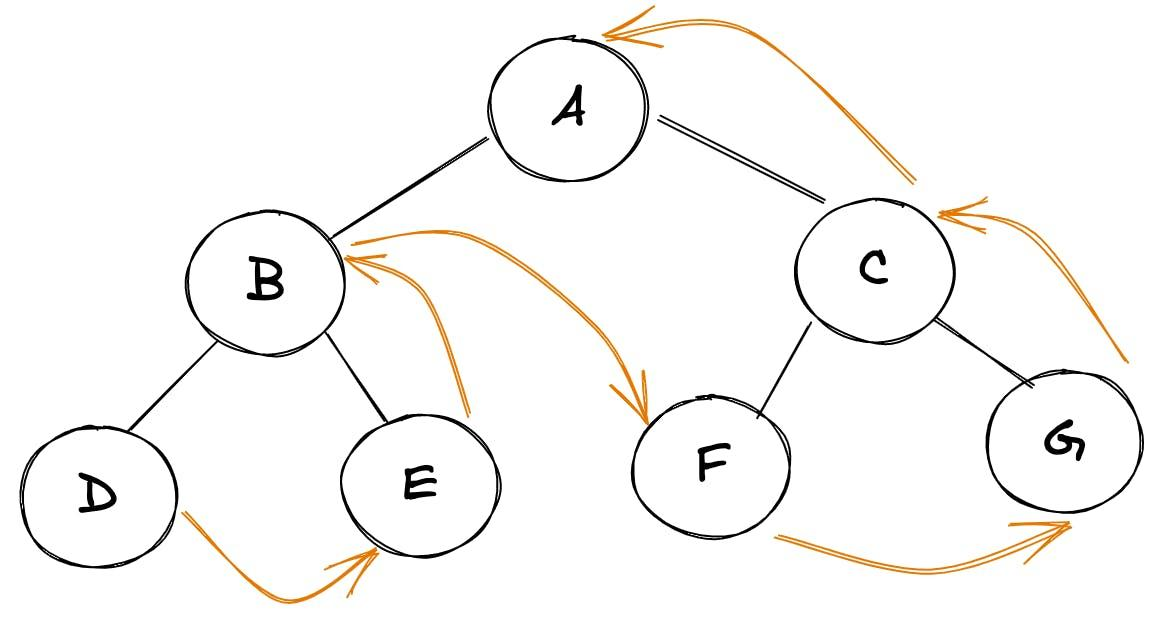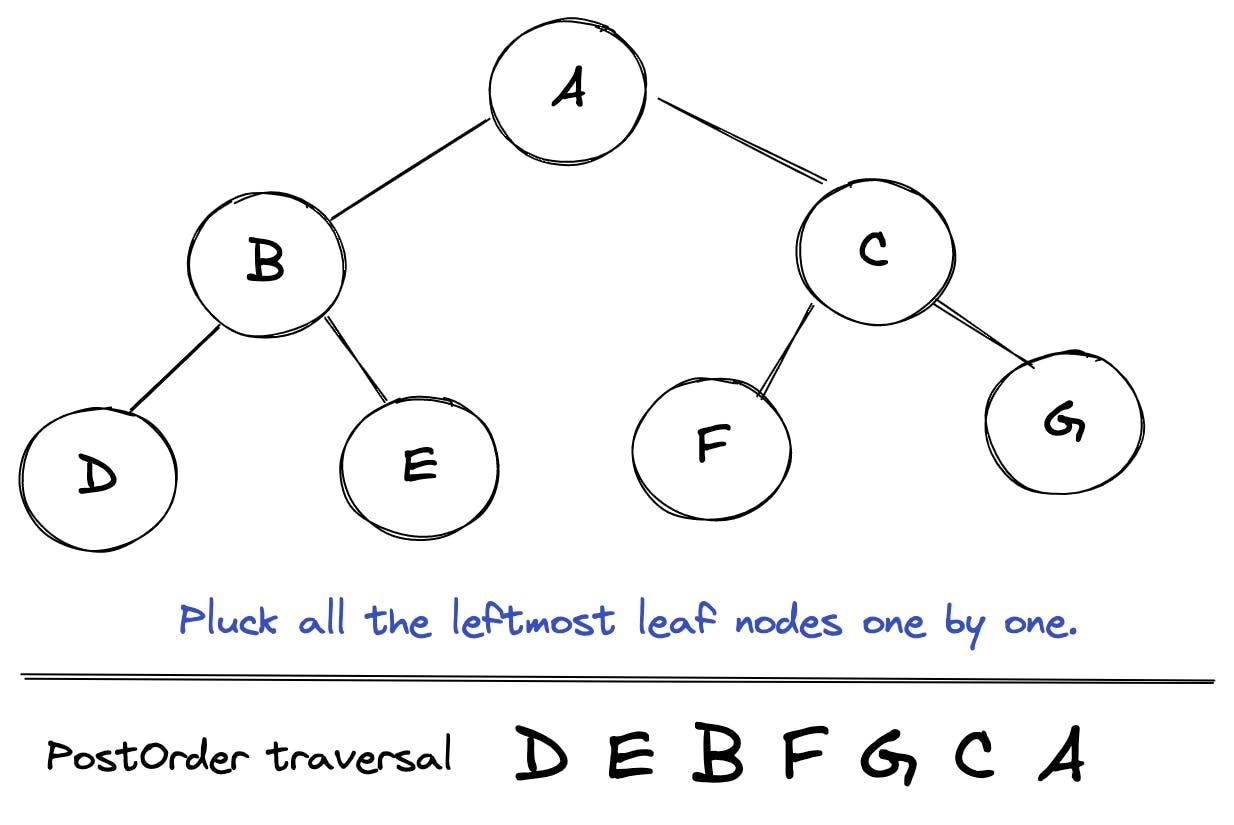```js function walkPostOrder(root){ if(root === null) return

if(root.left) walkPostOrder(root.left) if(root.right) walkPostOrder(root.right)

// do something here console.log(root.val)

} ```

```js // PreOrder: root -> left -> right

// Reverse of PreOrder: right -> left -> root

// But PostOrder is: left -> right -> root ```

```js // record result using root -> right -> left

// reverse result left -> right -> root ```

• 使用與上面的迭代前序演算法類似的方法，使用臨時`堆疊`
• 唯一的例外是我們使用 `root-> right-> left` 而不是 `root-> left-> right`

• 將遍歷序列記錄在一個數組`結果`

• `結果`的逆序給出了後序遍歷

```js function walkPostOrder(root){ if(root === null) return []

const tempStack = [root], result = []

while(tempStack.length){ const last = tempStack.pop()

``````  result.push(last)

if(last.left) tempStack.push(last.left)
if(last.right) tempStack.push(last.right)
}

return result.reverse()
``````

} ```

## 額外：JavaScript 提示

```js for(let node of walkPreOrder(tree) ){ console.log(node) }```

```js function* walkPreOrder(root){ if(root === null) return

const stack = [root] while(stack.length){ const item = stack.pop() yield item if(item.right) stack.push(item.right) if(item.left) stack.push(item.left) } } ```

「其他文章」